### Home > INT2 > Chapter 1 > Lesson 1.2.1 > Problem1-29

1-29.

Mei puts the polygons at right into a bucket and asks Brian to pick one.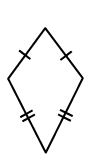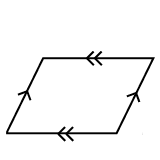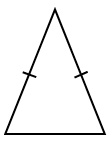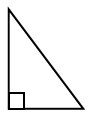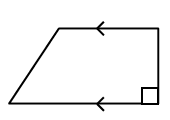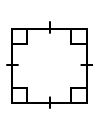1. What is the probability that he pulls out a quadrilateral with at least one pair of parallel sides?

Identify the shapes that are quadrilaterals with a $Q$.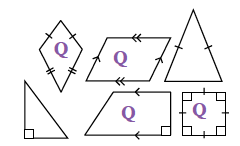Identify the shapes that have parallel sides with a $P$.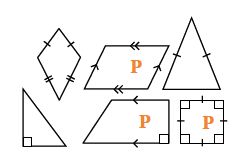Write a fraction where the numerator is the number of shapes that have both parallel sides and four sides, and where the denominator is the total number of shapes.

$\frac{3}{6}=\frac{1}{2}$

2. What is the probability that he pulls out a polygon with rotation symmetry?